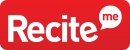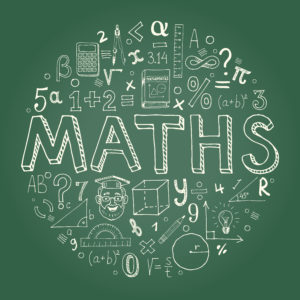For dyslexic students, the problem with word problems isn’t just the fact that they have to be read without error – but that there are many aspects of the language commonly found in word problems that will frustrate many dyslexic students if they aren’t explicitly taught where confusions arise.

Here are just a few language-based sources of difficult that arise in the reading of math word problems:

## #1. PASSIVE VOICE

Many students need to be explicitly taught about passive voice in grammar.

Math problems frequently lapse into passive voice without any attention to the differences that may occur in meaning.

For example, consider the differences in 8 divided by 2, 8 is divided into halves, and 8 is divided by half. In the first case, the answer is 4, whereas in the last, the answer is 16.

## #2. MULTIPLE WORD MEANINGS

There are many examples of multiple word meanings that occur in mathematics, and the different situations as well as ambiguous uses are best taught explicitly.

For example, words such as variable, function, plane, table, and even draw have different meanings in a mathematical context.

## #3. KEY WORD APPROACHES CAN BE MISLEADING

Although key word approaches can be helpful in some cases, zeroing in on certain key words in math problems can also lead students astray.

For example, “What must we add to 5 to obtain 13? With the key word approach, a student may circle the word “add” and then add 5 and 13 instead of subtracting.

Similarly: Jack has five dollars less than Jill. If Jack has seven dollars, how much does Jill have? The correct way to answer this problem is to add, but the key word approach might led a student to subtract.

The list of possible difficulties goes on and on for dyslexic students. The most important point to remember with dyslexic students is that if problems arise with math word problems, it may not be the math, ‘careless work’, or even reading problems per se. Tutors working with students should look carefully for language-based sources of confusion and explicitly teach distinctions in similar words.